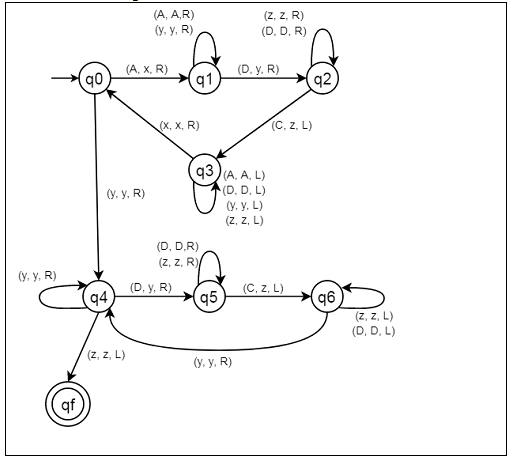# Construct a Turing machine for L = {aibjck | i< j< k; i ‚â• 1}

Here we will see how to make a Turing machine for language L = {AiBjCk | i < j < k; i ≥ 1}. So this represents a kind of language where we will use only three characters A, B and C. The w is a string. So if w = AABBBBCCCCC, The Turing machine will accept it.

To solve this, we will use this approach. Firstly compare two elements as a single element, after that comparing the single element if |first| > |(Second, Third)|, and |Second| > |Third|, then it will be accepted. Now if |Third| > |(First, Second)| and |First| > |Second|, then it will be accepted, otherwise not accepted.

## State Transition Diagram −